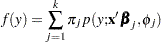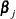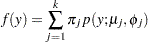### Homogeneous Mixtures

If the component distributions are of the same distributional form, the mixture is called homogeneous. In most applications of homogeneous mixtures, the mixing probabilities do not depend on regression parameters. The general model then simplifies toSince the component distributions depend on regression parameters, this model is known as a homogeneous regression mixture. A homogeneous regression mixture assumes that the regression effects are the same across the components, although the FMM procedure does not impose such a restriction. If the component distributions do not contain regression effects, the modelis the homogeneous mixture model. A classical case is the estimation of a continuous density as a-component mixture of normal distributions.